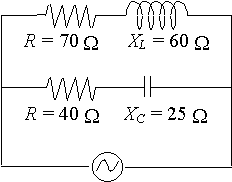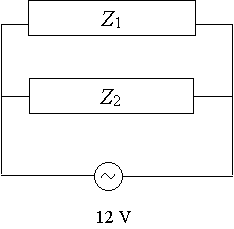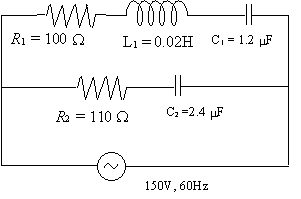Search IntMath
Close

# 12. Parallel AC Circuits

Recall Ohm's law for pure resistances:

V = IR

In the case of AC circuits, we represent the impedance (effective resistance) as a complex number, Z. The units are ohms (Ω).

In this case, Ohm's Law becomes:

V = IZ.

Recall also, if we have several resistors (R1, R2, R3, R4, …) connected in parallel, then the total resistance RT, is given by:

1/(R_T)=1/R_1+1/R_2+1/R_3+...

In the case of AC circuits, this becomes:

1/(Z_T)=1/Z_1+1/Z_2+1/Z_3+...

Continues below

## Simple case:

If we have 2 impedances Z1 and Z2, connected in parallel, then the total resistance ZT, is given by

1/(Z_T)=1/Z_1+1/Z_2

We can write this as:

1/(Z_T)=(Z_2+Z_1)/(Z_1Z_2)

Finding the reciprocal of both sides gives us:

Z_T=(Z_1Z_2)/(Z_1+Z_2)

### Example 1

Find the combined impedance of the following circuit:Call the impedance given by the top part of the circuit Z1 and the impedance given by the bottom part Z2.

We see that Z1 = 70 + 60j Ω and Z2 = 40 − 25j Ω

So

Z_T=(Z_1Z_2)/(Z_1+Z_2)

=((70+60j)(40-25j))/((70+60j)+(40-25j))

=((70+60j)(40-25j))/(110+35j)

(Adding complex numbers should be done in rectangular form.

Now, we convert everything to polar form and then multiply and divide as follows):

=((70+60j)(40-25j))/(110+35j)

=((92.20/_40.60^text(o))(47.17/_-32.01^text(o)))/(115.4/_17.65^text(o))

(We do the product on the top first.)

=((92.20xx47.17)/_(40.60^text(o)-32.01^text(o)))/(115.4/_17.65^text(o))

=(4349.074/_8.59^text(o))/(115.4/_17.65^text(o))

(Now we do the division.)

=(4349.074)/115.4/_(8.59^text(o)-17.65^text(o))

=37.69/_-9.06^text(o)

(We convert back to rectangular form.)

=37.22-5.93j

(When multiplying complex numbers in polar form, we multiply the r terms (the numbers out the front) and add the angles. When dividing complex numbers in polar form, we divide the r terms and subtract the angles. See the Products and Quotients section for more information.)

So we conclude that the combined impedance is

Z_T = 37-5.9j\ Omega

### Example 2

Given that Z1= 200 − 40j Ω and Z2= 60 + 130j Ω,find

a) the total impedance

b) the phase angle

c) the total line current

a) Z_T =frac{Z_1Z_2}{Z_1 + Z_2}

=frac{(200-40j)(60+130j)}{(200-40j)+(60+130j)}

=frac{(200-40j)(60+130j)}{260+90j}

=frac{(204.0angle-11.31^@)(143.2angle65.22^@)}{(275.1angle19.09^@)}

=frac{204.0times143.2}{275.1}angle(-11.31^@+ 65.22^@- {:19.09^@)

=106.2angle34.82^@

=87.18+60.64j

So we conclude that the total impedance is

Z_T = 87.2+60.6j\ Omega

b) We see from the second last line of our last answer that the phase angle is ~~35^@.

c) Total line current:

We use

• V = IZ,
• the fact that the impedance is 106.2 ∠ 34.82^@ and
• the fact that the voltage supplied is 12 V = 12 ∠ 0^@\ V.

So

I = V/Z

=frac{12angle0^@}{106.2angle34.82^@}

=0.113angle-34.82^@ "A"

### Example 3

A 100\ Ω resistor, a 0.0200\ "H" inductor and a 1.20\ mu"F" capacitor are connected in parallel with a circuit made up of a 110\ Ω resistor in series with a 2.40\ mu"F" capacitor. A supply of 150\ "V", 60\ "Hz" is connected to the circuit.

Calculate the total current taken from the supply and its phase angle.For Z1 (the upper part of the circuit), we have:

XL = 2πfL = 2π (60)(0.0200) = 7.540 Ω

X_C=1/(2pi(60)(1.20xx10^-6))

=2210.485\ Omega

Z1 = R1 + j(XLXC)

= 100 + j(7.540 - 2210.485)

= 100 − 2202.9j

= 2205.21 ∠ − 87.40^@\ Ω

For Z2 (the lower part of the circuit), we have:

X_C=1/(2pi(60)(2.40xx10^-6))

=1105.243\ Omega

Z2 = R2 + j(XL - XC)

= 110 + j(−1105.243)

= 1110.7 ∠ −84.32^@\ Ω

So the total impedance, ZT, is given by:

Z_T=(Z_1Z_2)/(Z_1+Z_2)

=(2449326.75/_-171.72^"o")/(210-3308.188j)

=(2449326.75/_-171.72^"o")/(3314.85/_-86.37^"o")

=738.9/_-85.35^"o"

This last line in rectangular form is ZT = 59.9 − 736.5j Ω

Now:

I_T=(V_T)/(Z_T)

=(150/_0^"o")/(738.9/_-85.35^"o")

=0.203/_85.35^"o"

So the total current taken from the supply is 203\ "mA" and the phase angle of the current is ~~85^@.

## Problem SolverThis tool combines the power of mathematical computation engine that excels at solving mathematical formulas with the power of GPT large language models to parse and generate natural language. This creates math problem solver thats more accurate than ChatGPT, more flexible than a calculator, and faster answers than a human tutor. Learn More.